#0
0
01. 云栖社区>
2. 博客>
3. 正文

## 异常检测概览——孤立森林 效果是最好的

### 用机器学习检测异常点击流• 数据预处理
• 病毒木马检测
• 工业制造产品检测
• 网络流量检测

### 二、异常检测算法

#### 1. 基于统计与数据分布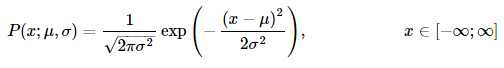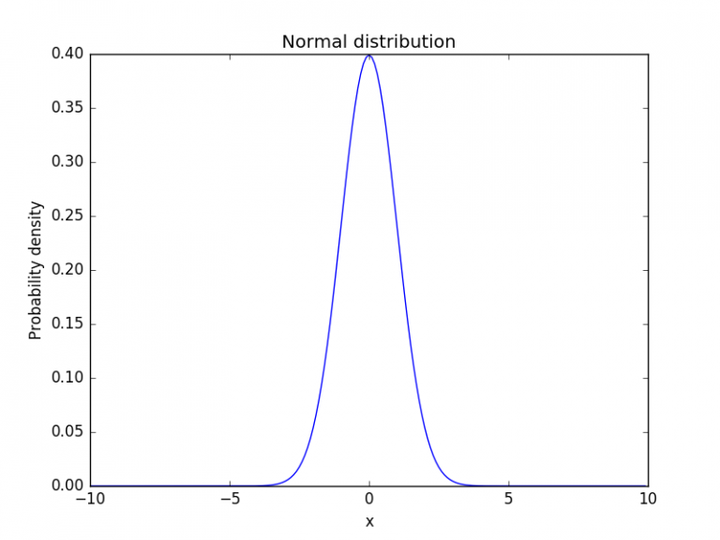``````import tushare
from matplotlib import pyplot as plt

df = tushare.get_hist_data("600680")
v = df[-90: ].volume
v.plot("kde")
plt.show()
``````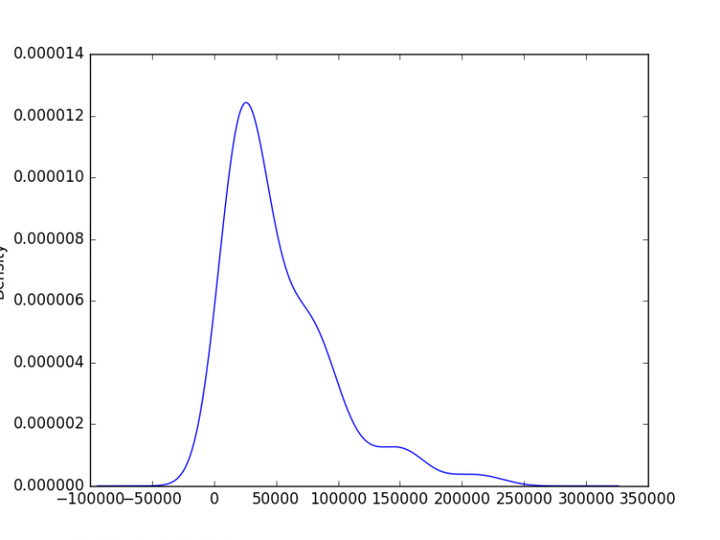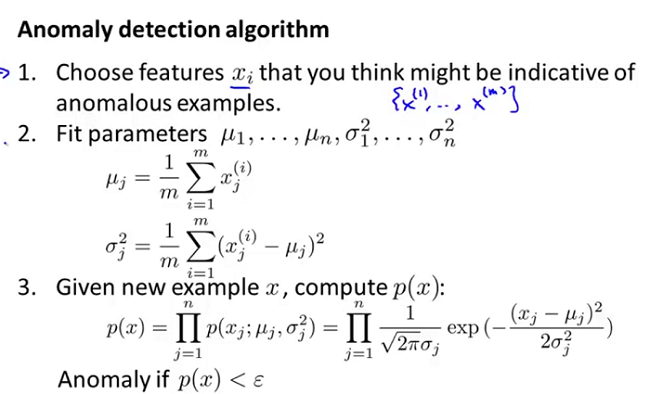#### 2. 箱线图分析

``````import tushare
from matplotlib import pyplot as plt

df = tushare.get_hist_data("600680")
v = df[-90: ].volume
v.plot("kde")
plt.show()
``````

``````import tushare
from matplotlib import pyplot as plt

df = tushare.get_hist_data("600680")
v = df[-90: ].volume
v.plot("kde")
plt.show()
``````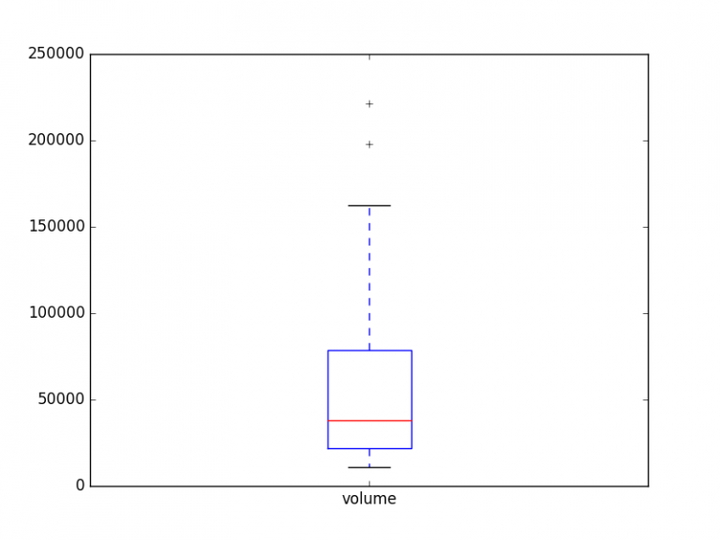#### 3. 基于距离/密度

4. 基于划分思想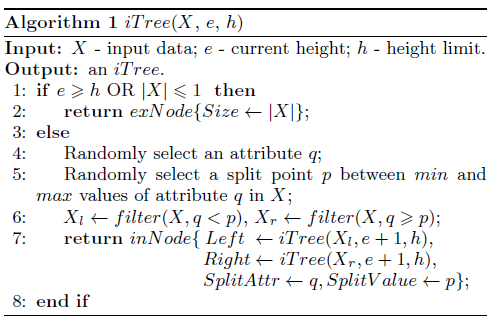``````import numpy as np
import matplotlib.pyplot as plt
from sklearn.ensemble import IsolationForest

rng = np.random.RandomState(42)

# Generate train data
X = 0.3 * rng.randn(100, 2)
X_train = np.r_[X + 1, X - 3, X - 5, X + 6]
# Generate some regular novel observations
X = 0.3 * rng.randn(20, 2)
X_test = np.r_[X + 1, X - 3, X - 5, X + 6]
# Generate some abnormal novel observations
X_outliers = rng.uniform(low=-8, high=8, size=(20, 2))

# fit the model
clf = IsolationForest(max_samples=100*2, random_state=rng)
clf.fit(X_train)
y_pred_train = clf.predict(X_train)
y_pred_test = clf.predict(X_test)
y_pred_outliers = clf.predict(X_outliers)

# plot the line, the samples, and the nearest vectors to the plane
xx, yy = np.meshgrid(np.linspace(-8, 8, 50), np.linspace(-8, 8, 50))
Z = clf.decision_function(np.c_[xx.ravel(), yy.ravel()])
Z = Z.reshape(xx.shape)

plt.title("IsolationForest")
plt.contourf(xx, yy, Z, cmap=plt.cm.Blues_r)

b1 = plt.scatter(X_train[:, 0], X_train[:, 1], c='white')
b2 = plt.scatter(X_test[:, 0], X_test[:, 1], c='green')
c = plt.scatter(X_outliers[:, 0], X_outliers[:, 1], c='red')
plt.axis('tight')
plt.xlim((-8, 8))
plt.ylim((-8, 8))
plt.legend([b1, b2, c],
["training observations",
"new regular observations", "new abnormal observations"],
loc="upper left")
plt.show()
``````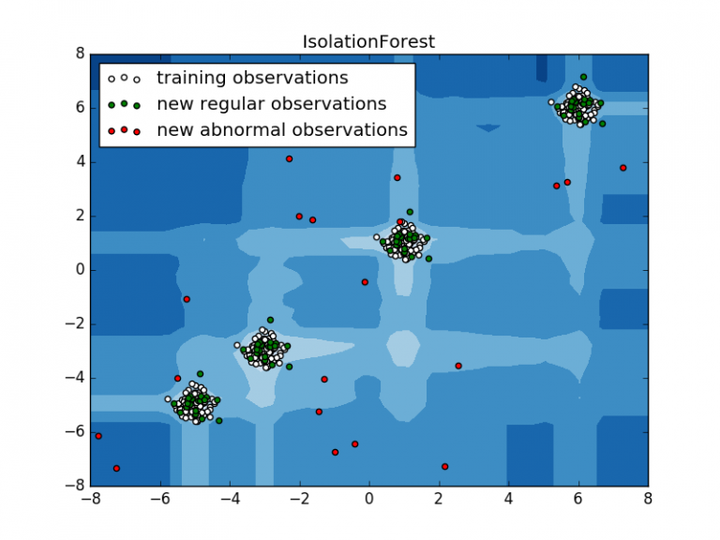+ 关注